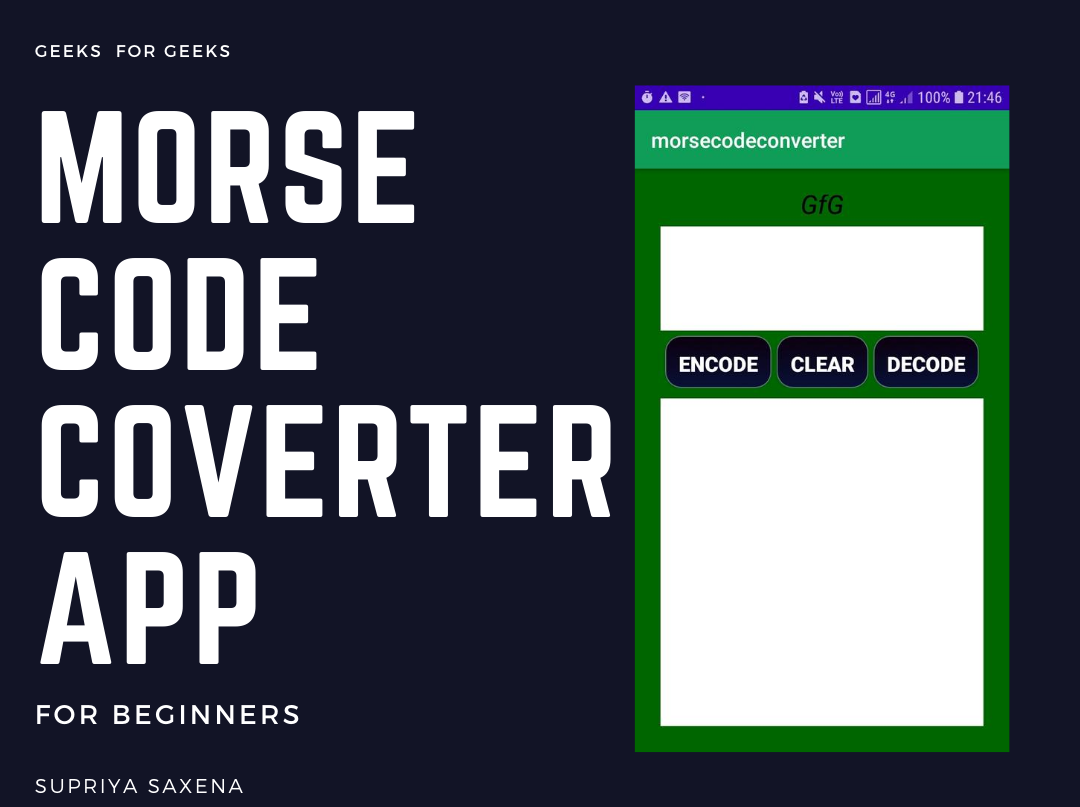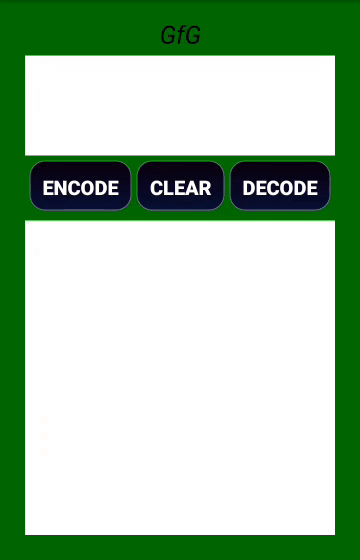Open in App
Not now

# How to Create a Morse Code Converter Android App?

• Difficulty Level : Hard
• Last Updated : 03 Nov, 2022

Morse code is a way in which one can encode characters and text using dots and dashes. The international Morse codes contain 26 letters of the alphabets and some non-English letters that are Arabic numerals as well as some punctuation. In Morse code, there is no difference between the upper and lower case letters. The transmission of the Morse Code is measured in dot durations. The Morse Code Converter App is an app that is used to convert the given statements to Morse Code. This is done by using Android Studio. The Languages that are used are Java and XML.So in this article lets create a Morse Code Converter Android App using the Java language. This project also involves the conversion of the Morse Code into relevant Statements. It means both encoding and decoding can be done using this Android app.#### Software Tools Required

The software tools required in this project are:

1. ANDROID-STUDIO IDE (1.0.2)
2. SDK having API level-21(minimum version)
3. JAVA 7 and above
4. An android smartphone – version 4.2.2(Jelly bean and above) (only for testing the software)

#### Approach

Step 1: Create a New Project To create a new project in Android Studio please refer How to Create/Start a New Project in Android Studio. Note that select Java as the programming language. Step 2: Create a Round Button This step is optional (only if you want a black button with round corners). In the project tab, in the left-hand corner of the screen, click on the app folder. Then, click on the res folder. Then, right-click on the drawable folder and select New then select Drawable Resource file. Give this resource file a name. Remember one can use only lower case letters for the name of the resource file. Write the code below to create a resource file for black buttons with round corners. Use this resource file as background in the XML code of buttons to get black buttons with round corners.

mybutton.xml

``````
<?xml version="1.0" encoding="utf-8"?>
<selector xmlns:android="http://schemas.android.com/apk/res/android">
<item>
<shape android:shape="rectangle">
<stroke android:width="1dip" android:color="#5e7974" />
android:startColor="#0C000E" />
</shape>
</item>
</selector>
``````

In case one wants to use the default button or some other button then skip this optional step and don’t include the resource file in the background of the buttons. Step 3: Working with activity_main.xml file

• Open the activity_main.xml file and start writing the xml code.
• Create 2 EditTexts in the xml file. One for writing the input and the other one for displaying the output. EditTexts are used because on clicking on the EditTexts the text can be selected and copied which will be very useful in sending the coded/uncoded message some other person using any messaging app.
• Make 3 Buttons: Encode, Decode and Clear.
• The Encode Button will take the input as text from the input EditText and then encode it into morse code and display it in the output EditText.
• The Decode Button will take the morse code as input from the input EditText and then decode it into alphabets and numbers and display it in the output EditText.
• The Clear Button will clear the input and output EditTexts.
• Give id’s to all the EditTexts and Buttons.

The complete xml code is given below:

activity_main.xml

``````
<?xml version="1.0" encoding="utf-8"?>
<RelativeLayout xmlns:android="http://schemas.android.com/apk/res/android"
xmlns:tools="http://schemas.android.com/tools"
android:layout_width="match_parent"
android:layout_height="match_parent"
android:background="#006600"
tools:context=".MainActivity">

<TextView
android:id="@+id/tvgfg"
android:layout_width="match_parent"
android:layout_height="wrap_content"
android:layout_marginTop="-8dp"
android:gravity="center"
android:text="GfG"
android:textAlignment="center"
android:textColor="#000"
android:textSize="25sp"
android:textStyle="italic" />

<EditText
android:id="@+id/etinput"
android:layout_width="match_parent"
android:layout_height="100dp"
android:layout_below="@+id/tvgfg"
android:layout_marginTop="5dp"
android:background="#ffffff"
android:gravity="start" />

<!--edit text to accept the input from the user-->
<LinearLayout
android:id="@+id/llout"
android:layout_width="match_parent"
android:layout_height="50dp"
android:layout_below="@+id/etinput"
android:layout_marginTop="5dp"
android:gravity="center"
android:orientation="horizontal">

<Button
android:id="@+id/btnencode"
android:layout_width="wrap_content"
android:layout_height="match_parent"
android:layout_marginRight="5dp"
android:background="@drawable/mybutton"
android:text="EnCode"
android:textColor="#fff"
android:textSize="20sp"
android:textStyle="bold" />

<Button
android:id="@+id/btnclear"
android:layout_width="wrap_content"
android:layout_height="match_parent"
android:layout_marginRight="5dp"
android:background="@drawable/mybutton"
android:text="clear"
android:textColor="#fff"
android:textSize="20sp"
android:textStyle="bold" />

<Button
android:id="@+id/btndecode"
android:layout_width="wrap_content"
android:layout_height="match_parent"
android:background="@drawable/mybutton"
android:text="decode"
android:textColor="#fff"
android:textSize="20sp"
android:textStyle="bold" />
</LinearLayout>

<!--edit text to display output to the user.
Edit text is used since the user can copy the
text easily if he wants to-->
<EditText
android:id="@+id/etoutput"
android:layout_width="match_parent"
android:layout_height="match_parent"
android:layout_below="@+id/llout"
android:layout_marginTop="10dp"
android:background="#ffffff"
android:gravity="start"
android:textSize="20sp"
android:textStyle="bold" />

</RelativeLayout>
``````

Step 4: Working with MainActivity.java file

• Open the MainActivity.java file.
• Initialize the variables just under the MainActivity class (Syntax:- Edit Text etinput, etoutput;Button btnEncode, btnDecode, btnclear;)
• Assign the variables under the OnCreate method.
• Make an array and store all the alphabets and digits in it.
• Make another array and store the morse code of each alphabet and digits in the indices corresponding to it’s alphabets and digits in the first array.
• Use the logic in the code below to complete the morse code converter app.

Below is the complete code for MainActivity.java file.

MainActivity.java

``````
package com.example.morseconverter;

import android.os.Bundle;
import android.view.View;
import android.widget.Button;
import android.widget.EditText;
import androidx.appcompat.app.AppCompatActivity;

public class MainActivity extends AppCompatActivity {

// initialize variables
EditText etinput,
etoutput;
Button btnEncode,
btnDecode,
btnclear;

@Override
protected void onCreate(Bundle savedInstanceState) {
super.onCreate(savedInstanceState);
setContentView(R.layout.activity_main);

// Assign variables
etinput = findViewById(R.id.etinput);
etoutput = findViewById(R.id.etoutput);
btnDecode = findViewById(R.id.btndecode);
btnEncode = findViewById(R.id.btnencode);
btnclear = findViewById(R.id.btnclear);

// initializing string arrays
final String[] AlphaNumeric = new String;

// string array for storing alphabets and numbers
final String[] AlphaNumeric1 = new String;

// string array for storing corresponding morse code
// assigning alphabets to the string array Alphanumeric[]
AlphaNumeric = "A";
AlphaNumeric = "B";
AlphaNumeric = "C";
AlphaNumeric = "D";
AlphaNumeric = "E";
AlphaNumeric = "F";
AlphaNumeric = "G";
AlphaNumeric = "H";
AlphaNumeric = "I";
AlphaNumeric = "J";
AlphaNumeric = "K";
AlphaNumeric = "L";
AlphaNumeric = "M";
AlphaNumeric = "N";
AlphaNumeric = "O";
AlphaNumeric = "P";
AlphaNumeric = "Q";
AlphaNumeric = "R";
AlphaNumeric = "S";
AlphaNumeric = "T";
AlphaNumeric = "U";
AlphaNumeric = "V";
AlphaNumeric = "W";
AlphaNumeric = "X";
AlphaNumeric = "Y";
AlphaNumeric = "Z";
AlphaNumeric = "0";
AlphaNumeric = "1";
AlphaNumeric = "2";
AlphaNumeric = "3";
AlphaNumeric = "4";
AlphaNumeric = "5";
AlphaNumeric = "6";
AlphaNumeric = "7";
AlphaNumeric = "8";
AlphaNumeric = "9";
AlphaNumeric = " ";

// assigning the corresponding morse code
// for each letter and number to
// Alphanumeric1[] array
AlphaNumeric1 = ".-";
AlphaNumeric1 = "-...";
AlphaNumeric1 = "-.-.";
AlphaNumeric1 = "-..";
AlphaNumeric1 = ".";
AlphaNumeric1 = "..-.";
AlphaNumeric1 = "--.";
AlphaNumeric1 = "....";
AlphaNumeric1 = "..";
AlphaNumeric1 = ".---";
AlphaNumeric1 = "-.-";
AlphaNumeric1 = ".-..";
AlphaNumeric1 = "--";
AlphaNumeric1 = "-.";
AlphaNumeric1 = "---";
AlphaNumeric1 = ".--.";
AlphaNumeric1 = "--.-";
AlphaNumeric1 = ".-.";
AlphaNumeric1 = "...";
AlphaNumeric1 = "-";
AlphaNumeric1 = "..-";
AlphaNumeric1 = "...-";
AlphaNumeric1 = ".--";
AlphaNumeric1 = "-..-";
AlphaNumeric1 = "-.--";
AlphaNumeric1 = "--..";
AlphaNumeric1 = "-----";
AlphaNumeric1 = ".----";
AlphaNumeric1 = "..---";
AlphaNumeric1 = "...--";
AlphaNumeric1 = "....-";
AlphaNumeric1 = ".....";
AlphaNumeric1 = "-....";
AlphaNumeric1 = "--...";
AlphaNumeric1 = "---..";
AlphaNumeric1 = "----.";
AlphaNumeric1 = "/";

btnEncode.setOnClickListener(new View.OnClickListener() {@Override
public void onClick(View v) {

// When button encode is clicked then the
// following lines inside this curly
// braces will be executed

// to get the input as string which the user wants to encode
String input = etinput.getText().toString();

String output = "";

// variable used to compute the output
// to get the length of the input string
int l = input.length();

// variables used in loops
int i, j;

for (i = 0; i < l; i++) {

// to extract each Token of the string at a time
String ch = input.substring(i, i + 1);

// the loop to check the extracted token with
// each letter and store the morse code in
// the output variable accordingly
for (j = 0; j < 37; j++) {

if (ch.equalsIgnoreCase(AlphaNumeric[j])) {

// concat space is used to separate
// the morse code of each token
output = output.concat(AlphaNumeric1[j]).concat(" ");

}
}
}

// to display the output
etoutput.setText(output);
}
});
btnclear.setOnClickListener(new View.OnClickListener() {@Override
public void onClick(View v) {
// When button clear is clicked then the
// following lines inside this curly
// braces will be executed

// to clear the etinput
etinput.setText("");

// to clear etoutput
etoutput.setText("");
}
});
btnDecode.setOnClickListener(new View.OnClickListener() {@Override
public void onClick(View v) {
// When button decode is clicked then the
// following lines inside this curly
// braces will be executed

// to get the input given by the user as string
String input1 = etinput.getText().toString();

// to add space to the end of the string
// because of the logic used in decoding
String input = input1.concat(" ");

// to get the length of the input string
int l = input.length();

// i and j are integer variables used in loops.
// Variable p is used as the end index of
// substring() function
int i, j, p = 0;

// variable used as a starting
// index of substring() function
int pos = 0;

// to store the extracted morse code
// for each Alphabet,number or space
String letter = "";

// a to store the output in it
String output = "";

for (i = 0; i < l; i++) {

// a variable used to trigger the j loop only
// when the complete morse code of a letter
// or number is extracted
int flag = 0;

// to extract each token at a time
String ch = input.substring(i, i + 1);

// if the extracted token is a space
if (ch.equalsIgnoreCase(" ")) {

// to store the value of i in p
p = i;

// to extract the morse code for each letter or number
letter = input.substring(pos, p);

// to update the value of pos so that next
// time the morse code for the next letter
// or digit is extracted
pos = p + 1;

flag = 1;
}
String letter1 = letter.trim();
// to delete extra whitespaces at
// both ends in case there are any
if (flag == 1) {
for (j = 0; j <= 36; j++) {
if (letter1.equalsIgnoreCase(AlphaNumeric1[j])) {
output = output.concat(AlphaNumeric[j]);
break;
}
}
}
}
// to display the output
etoutput.setText(output);
}
});
}
}
``````